# 1.学习总结

## 1.1图的思维导图# 2.PTA实验作业

## 题目1：7-3 六度空间

### 设计思路

    BFS(int v,int n,int e){
count表示六度空间访问点个数，last记录当前层最后一个元素
初始化visited数组
visited[v] = 1表示当前点已访问
初始化front = rear = -1 队列为空
V入栈
last = v记录当前层最后的点
while(队列不空)
V = 队首元素
for (i = 1 to i = n)
if(i未访问&&i于v点有边)
i入栈，visited[i] = 1，tail = i,count++
end if
if (v是单前层最后一个元素)level++,last = tail
end if
end while
return count


### 代码截图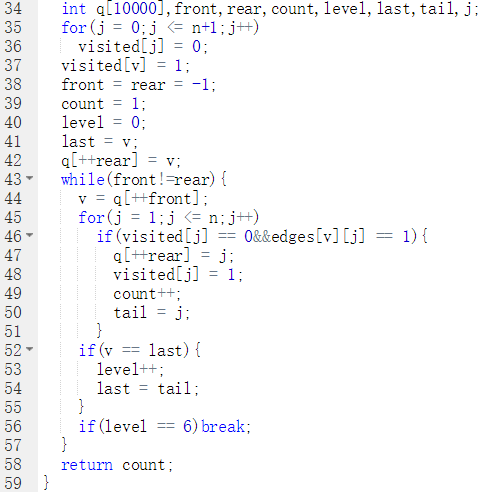### PTA提交列表说明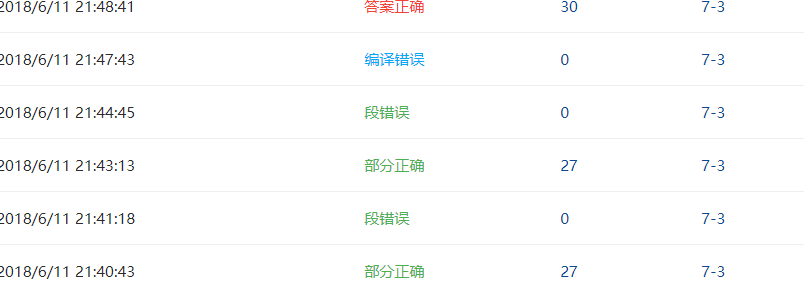## 题目2：7-4 公路村村通

### 设计思路

int prim(MGraph g){
lowcost数组记录和其他点最近距离,cost记录总成本
lowcost = 0出发点本身距离不存在，记为0
for(i = 2 to i = g.n)
lowcost[i] = g.edges[i]初始点的最近距离
end for
for(i = 2 to i= g.n)
min = 100001
while(j<=g.n)
if(路径存在&&路径<min)
min = lowcost[j];
k = j;
if(找不到最近的点)return -1
cost+=min
lowcost[k] = 0表示k点加入该集合
修正lowcost数组判断新加入的点是否有
与未加入集合点更近的
}


### 代码截图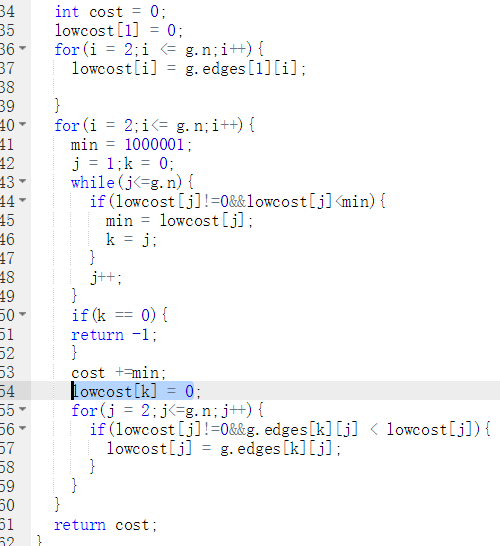### PTA提交列表说明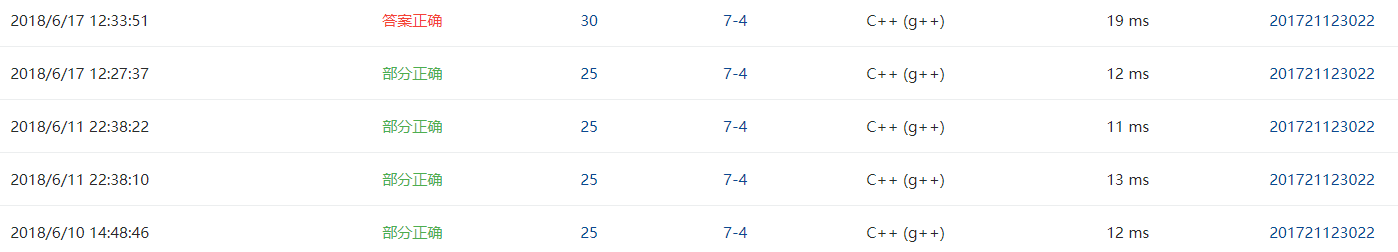## 题目3：7-7 旅游规划

### 设计思路

void Dijkstra(int v,int n,int e,int end){
定义dist数组存放距离path数组存放路径cost数组存放消费
定义s数组存放加入集合的点
先初始化这几个数组
将V加入s数组，path[v] = 0
mindis = INF;
for(j = 0 to j < n)
if(j未加入集合且dist[j]存在)
u = j;
mindis = dist[j];
end if
end for
s[u] = 1;
for(j = 0 to j < n)
if(j加入 集合)
if(新加入点距离比原来近)
更新dist[j],path[j],cost[j]
else if(新加入点距离和原来一样近但比较便宜)
更新dist[j],path[j],cost[j]


### 代码截图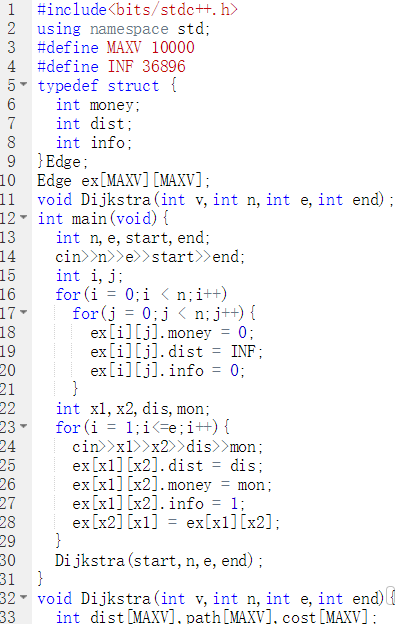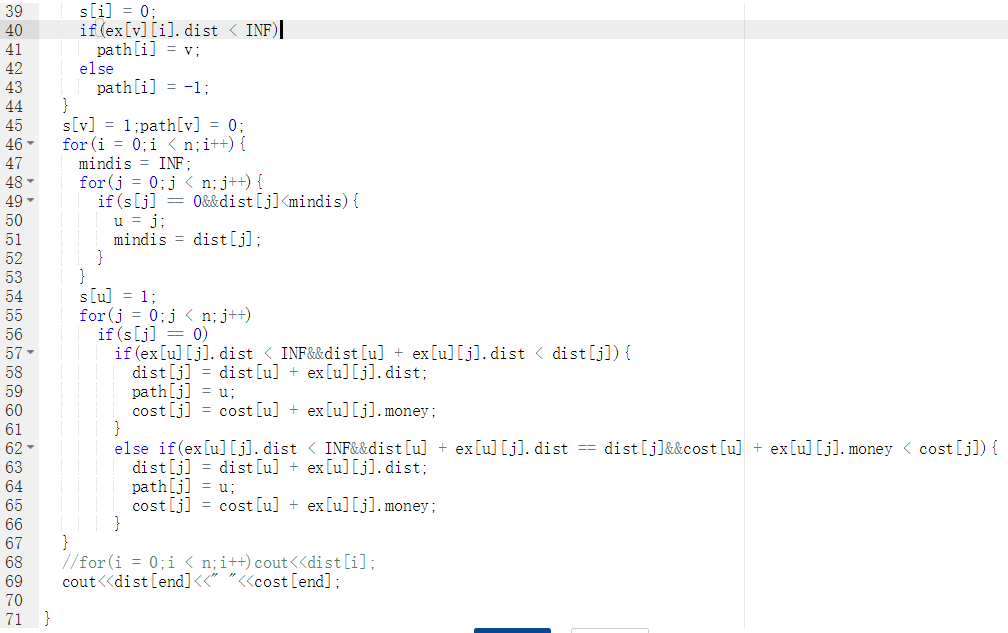### PTA提交列表说明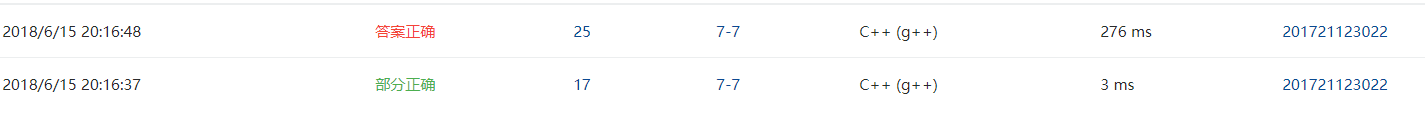# 3.截图本周题目集的PTA最后排名

## 3.1 PTA排名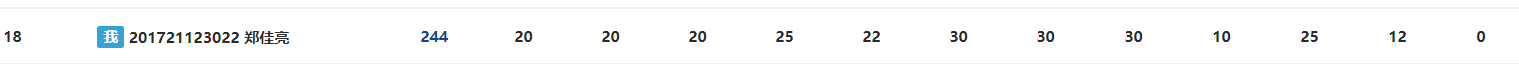# 4. 阅读代码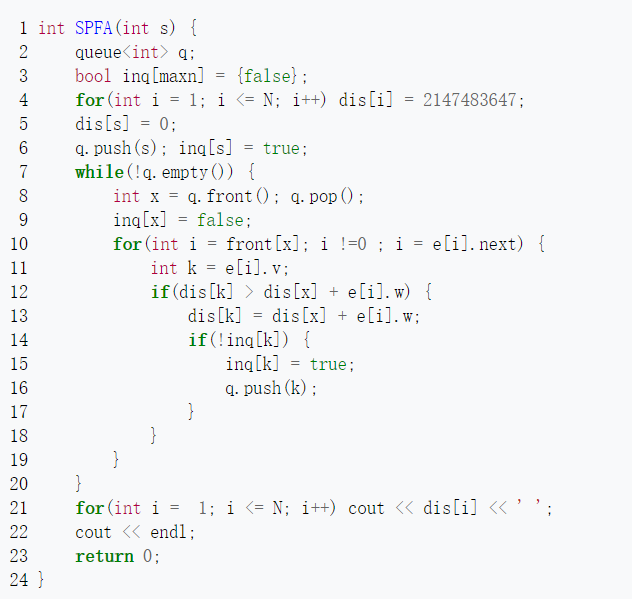posted @ 2018-06-17 20:17  Airoure  阅读(295)  评论(0编辑  收藏  举报